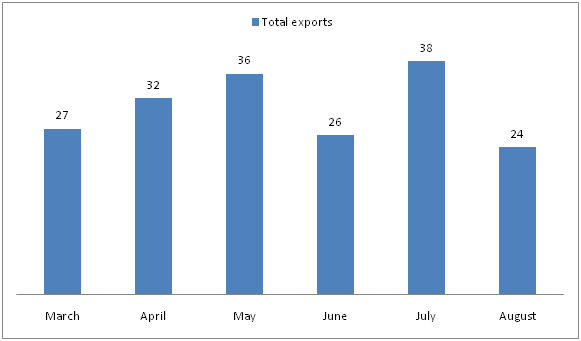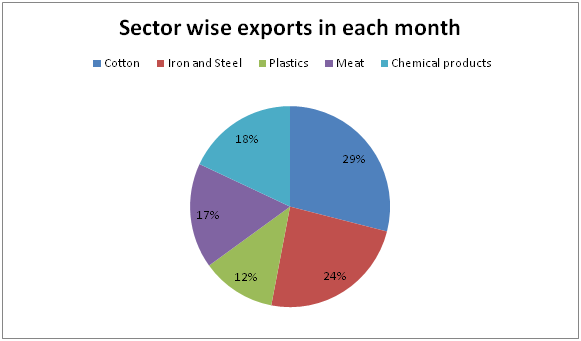# NIACL AO Mains– Quantitative Aptitude Questions Day- 19

Dear Readers, Bank Exam Race for the Year 2019 is already started, To enrich your preparation here we have providing new series of Practice Questions on Quantitative Aptitude – Section. Candidates those who are preparing for NIACL AO Mains 2019 Exams can practice these questions daily and make your preparation effective.

[WpProQuiz 5269]

Directions (1 – 5): What approximate value should come in the place of question mark (?) in the following questions?

1) 90.05 + 281 ÷ 4 – 151.21 = x

a) 512

b) 343

c) 1000

d) 729

e) 216

2) (17.98)2 ÷ (3.05)2 × 90.11 ÷ 5.01 = ? – 551 ÷ 3.98

a) 830

b) 720

c) 790

d) 880

e) 940

3) (5/7) of 341 + (2/3) of (7/15) of 1889 = ? % of 700

a) 154

b) 93

c) 119

d) 206

e) 250

4) √? + 27.65 = (375.39 + 72.01) ÷ 49.78 + (18.78 × 2.75)

a) 1225

b) 1444

c) 1681

d) 961

e) 2025

5) 36 % of 489.8 + 52 % of 301.25 = 4 × ?

a) 75

b) 66

c) 92

d) 127

e) 83

Directions (6 – 10): Study the following graph carefully and answer the given question.

The following bar graph shows total exports of India (In billion dollars) in different months in the year 2017.6) Find the average export of Cotton in the period of March to August?

a) 7.627 billion dollars

b) 8.845 billion dollars

c) 9.123 billion dollars

d) 6.452 billion dollars

e) None of these

7) Total export of Plastics and Chemical products in the month of May and June together is approximately what percentage of total export of Iron and steel and Meat in the month of July and August together?

a) 85 %

b) 96 %

c) 73 %

d) 108 %

e) 115 %

8) Find the difference between the total exports of Meat in the month of March, May and July together to that of total exports of Iron and Steel in the month of April, June and August together?

a) 2.51 billion dollars

b) 3.87 billion dollars

c) 4.26 billion dollars

d) 1.94 billion dollars

e) None of these

9) Find the ratio between the total exports of Cotton in the month of June and August together to that of total exports of Chemical products in the month of March and April together?

a) 412 : 245

b) 349 : 178

c) 623 : 452

d) 899 : 531

e) None of these

10) Total exports of Cotton and Meat in the month of March and August together is approximately what percentage more/less than the total exports of Iron and Steel and Chemical products in the month of April and June together?

a) 8 % more

b) 4 % more

c) 4 % less

d) 8 % less

e) 12 % more

Direction (1-5) :

90 + (280/4) – 151 = ∛x

90 + 70 – 151 = ∛x

9 = ∛x

X = 93 = 729

182 ÷ 32 × 90 ÷ 5 = x – (552/4)

[(18*18)/(3*3)]*(90/5) = x – (552/4)

648 + 138 = x

X = 786 = 790

(5/7) of 343 + (2/3) of (7/15) of 1890 = x % of 700

(5/7) * 343 + (2/3) * (7/15) * 1890 = (x/100)*700

245 + 588 = 7x

833 = 7x

X = 833/7

X = 119

√x + 28 = (375 + 72) ÷ 50 + (19 × 3)

√x + 28 = 447/50 + 57

√x = 9 + 57 – 28

√x = 38

X = 382 = 1444

36 % of 490 + 52 % of 300 = 4 × ?

(36/100)*490 + (52/100)*300 = 4x

4x = 176.4 + 156

4x = 332.4

X = (332.4/4) = 83.1

X = 83

Direction (6-10) :

The total export of Cotton in the period of March to August

= > [27 + 32 + 36 + 26 + 38 + 24]*(29/100)

= > 183*(29/100)

= > 53.07 billion dollars

Required average = 53.07/6 = 8.845 billion dollars

Total export of Plastics and Chemical products in the month of May and June together

= > 36*(30/100) + 26*(30/100)

= > 10.8 + 7.8

= > 18.6 billion dollars

Total export of Iron and steel and Meat in the month of July and August together

= > 38*(41/100) + 24*(41/100)

= > 15.58 + 9.84

= > 25.42 billion dollars

Required % = (18.6/25.42)*100 = 73.17 % = 73 %

The total exports of Meat in the month of March, May and July together

= > (27 + 36 + 38)*(17/100)

= > 17.17 billion dollars

The total exports of Iron and Steel in the month of April, June and August together

= > (32 + 26 + 24)*(24/100)

= > 19.68 billion dollars

Required difference = 19.68 – 17.17 = 2.51 billion dollars

The total exports of Cotton in the month of June and August together

= > (38 + 24)*(29/100)

= > 17.98 billion dollars

The total exports of Chemical products in the month of March and April together

= > (27 + 32)*(18/100)

= > 10.62 billion dollars

Required ratio = 17.98 : 10.62 = 899 : 531

Total exports of Cotton and Meat in the month of March and August together

= > (27 + 24)*[(29 + 17)/100]

= > 23.46 billion dollars

Total exports of Iron and Steel and Chemical products in the month of April and June together

= > (32 + 26)*[(24 + 18)/100]

= > 24.36 billion dollars

Required %

= > [(24.36 – 23.46)/24.36]*100 = 3.69 %

= > 4 % less